# Reaction Kinetics : Study of Rates of Chemical Processes.

Chemical kinetics, also known as Reaction kinetics, is the study of rates of chemical processes. The rate of a chemical reaction is, perhaps, its most important property because it dictates whether a reaction can occur during a lifetime. Knowing the rate law, an expression relating the rate to the concentrations of reactants can help a chemist adjust the reaction conditions to get a more suitable rate. If there are two competing reactions for a single reagent, one can, knowing the rate law, favor the exclusive formation of a single product.To obtain this kind of knowledge about reactions, we will first define what rate means. We will then derive the rate law expression. Using the method of initial rates, we will discuss how to determine the form and order of the rate law. Next, we will probe rate laws in depth and introduce the integrated rate law as an alternative form of the simple rate law that allows us another, more simple, experimental method to determine the first order reaction kinetics of the rate law. The integrated rate law will also allow us to determine the half-lives of chemical reactions.

## Fundamentals of Rate Laws

### Defining Rates

In order to introduce the idea of rate, let’s suppose we wish to know how fast the following reaction is going:

One way to do this is to define rate as the change in concentration of some species with respect to time, and then measure the concentrations of all species at multiple times to determine the rate. The results of such a hypothetical experiment are given in the for the reaction of hydrogen and iodine.

The initial concentrations of H2 and I2 are equal at all times and the initial concentration of product is zero:

Figure %: Graph of concentration versus time for the reaction of hydrogen and Iodine

As you can see, the rate of formation of HI is twice the rate of disappearance of H2 or I2 at any given time. Also, note that the rate slows in time due to decreasing concentrations of the reactants. Stated mathematically, the relationship between the formation of products and the disappearance of reactants for this reaction is: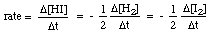In general, for the reaction below:

aA  +   bB   ->   cC   +   dD

The rate is expressed as follows: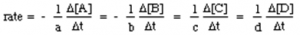Another expression for a rate is called the differential rate law, or simply, the rate law. It expresses the rate of a reaction in terms of the concentrations of the reactants raised to an experimentally determined power. The exponent on each concentration term is called the order of the reaction in that particular reactant.

The sum of the exponents in the rate law is called the order of the reaction. The powers on the concentration terms in a rate law are NOT the stoichiometric coefficients from the balanced equation! For example, the rate law for the will have the following form: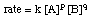Note that the exponents are not a and b but some experimentally determined powers p and q which may or may not equal a and b. The order of the is, therefore, p + q. We will discuss in Determining Rate Laws how those exponents can be determined.

Also, notice in the presence of the rate constant k. Students often have trouble distinguishing between the rate of a reaction and its rate constant. The rate of a reaction is the total rate of a reaction and is the “rate” in the rate law.

The units of rate are always M / s. The rate constant, k, is an experimentally determined proportionality constant that gives some measure of the intrinsic “reactivity” of the reaction. The units on the rate constant depend on the order of the reaction, n, such that k has units M1 – n / s.

In that way, the units of the rate constant, k, are chosen to make the units of rate always M / s regardless of order. For example, if the order of the reaction is 3, the rate law could be rate = k [A]2[B]. The units of rate are M / s and the rate constant must, therefore, have units of M-2 / s.

The attentive reader may have noticed that we have only considered the rate of the forward reaction, neglecting any sort of reverse reaction in the rate law. To make our math easier, we have intentionally ignored the reverse reaction and we will continue to do so. This is a justified practice for reactions with negligible reverse rates, such as those with equilibrium constants, K, much greater than 1. For reactions with K’s around 1, this is also a valid approximation because they are usually carried out such that products are removed from the reaction mixture as they are formed, keeping concentrations of products low.

Rate laws can also be expressed to relate the concentration of reactants to the time of the reaction. Such an expression is called an integrated rate law because it is the integral of the differential rate law. For those of you who have had some calculus, we present a derivation of an integrated rate law from the differential rate law below for a first-order reaction. If you have not had calculus, don’t worry. Just pay attention to the end result of the derivation.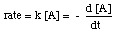Rearranging this equation gives the following: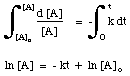Using a similar technique, one can derive the integrated rate law for any differential rate law. As we will see in Determining Rate Laws, the fact that the 1st order integrated rate law is linear allows us to test if a reaction is first order by plotting ln [A] versus time.

## Determining the Rate Law

### Kinetics Experiments:

The goal of a kinetics experiment is to measure the concentration of a species at a particular time during a reaction so that a rate law can be determined. However, it is exceedingly difficult to get an accurate measurement of a concentration at a known time because the techniques used to measure concentrations don’t work instantaneously, but rather take time to perform.

One of the earliest methods used to measure concentrations at specified times is to quench the reaction either by flash freezing it or by adding a substance that severely inhibits the reaction. Both of these techniques are problematic because one can’t be sure that the reaction has completely stopped.

The reaction may still be going on during the analysis. Additionally, the reaction mixture is destroyed for the purposes of kinetic experiments, so the chemist must make multiple trial runs and waste a lot of reagents to observe the concentrations at multiple points in time.

A more modern technique to measure concentration is absorbance spectroscopy. This experiment may be used when a product or reactant has an absorbance frequency unique to those of other components of the reaction mixture.

By measuring the absorbance of a particular product or reactant at a variety of known concentrations, you can construct a plot of absorbance versus concentration called a Beer’s Law plot. This calibration chart allows you to calculate the unknown concentration given the reaction solution’s absorbance. The advantage of this method is that a large number of data points with well-known times can be quickly collected using only one reaction mixture.

## Method of Initial Rates

When looking at the expression for them, you should notice that the variables in the equation are the concentration terms and the powers p and q:Because we can measure the concentrations in the rate law using the techniques described above, the unknowns we wish to measure are k, p, and q. One method of directly measuring k, p, and q are called the method of initial rates. By measuring the initial rate (the rate near reaction time zero) for a series of reactions with varying concentrations, we can deduce to what power the rate depends on the concentration of each reagent. For example, let’s use the method of initial rates to determine the rate law for the following reaction: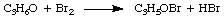whose rate law has the form: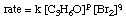Using the following initial rates data, it is possible to calculate the order of the reaction for both bromine and acetone:

Figure %: Initial rates data for the bromination of acetone

To calculate the order of the reaction for bromine, notice that experiments 1 and 2 hold the concentration of acetone constant while doubling the concentration of bromine. The initial rate of the reaction is unaffected by the increase in bromine concentration, so the reaction is zero order in bromine. We can prove this mathematically by taking the ratio of the rates from experiments 1 and two: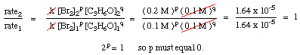As you can see in the above equations, by holding the concentrations of all but one species constant between two experiments, you can calculate the order of the reaction in a single reactant at a time. By similar reasoning, we can conclude that because the rate of reaction doubled when the concentration of acetone was doubled (cf. experiments 1 and 3) the reaction must be first order in acetone.

However, had the rate quadrupled or coupled with a doubling of the acetone concentration, the reaction would have been second or third order in acetone, respectively. In practice, you will likely never see a reaction with an order higher than 3.

If you calculate an order higher than 3 for a reaction, double check your math because that is highly unusual. If you compute a fractional power for a reactant’s order, do not be discouraged; they are quite common (especially half-order reactions).

To calculate the value of k, the rate constant, you simply plug into the rate law the values of the concentrations, the orders, and the rate of the reaction from any one of the three experiments. All three experiments should give a value of 1.64 x 10-4 s-1. You should prove this to yourself.

## Using the Integrated Rate Law to Determine the Rate Law

One major disadvantage of using the method of initial rates is the need to perform multiple experiments. Another disadvantage is that it works only for relatively slow reactions. If your reaction proceeds too fast, the rate that you measure will have a large range of uncertainty.

To combat these problems, chemists have developed a method that uses data on concentration versus time during a reaction to infer the order of a reaction. The strategy works by comparing concentration versus time information to the mathematical predictions made by integrated rate laws.

This is usually accomplished by assuming that a reaction has a certain order and making a plot out of the data that should be linear if the assumption about the rate law is correct. If we guess correctly, the graph is linear. If we are wrong, then the graph is curved and we need to choose another order against which to plot our data.

To practice this method, we will first need to know the forms of the integrated rate laws for some common reaction orders. Luckily, only orders zero through two are common so we need to only consider reactions with these three orders.

Due to the mathematical complexities of the problem, we will only consider rate laws of the formation rate = [A]n. However, with some experimental tricks, I will explain below, that treatment does allow us to use the method of integrated rates for a reaction with any arbitrary rate law.

By integrating the differential rate law (the simple rate law from Heading ), we derive the integrated rate law for a reaction. Without proof, we present below the forms of the integrated rate laws for reaction orders zero through two:

Figure %: Integrated rate laws for reaction orders zero through two

As you can see, each order of reaction has a unique input and output variable that produces a straight line. For example, if we graph the following rate data for the decomposition of H2O2 assuming that it could be zero, first, or second order, we find that only the graph for a 2nd order reaction (1/[A] versus t) gives a straight line. Therefore, the reaction has the rate law rate = k [H2O2]2.

Assuming that we did not know that the decomposition is second order, we will make a series of three graphs to determine the order of the reaction:

Figure 1: Plot of Hydrogen Peroxide Concentration versus Time

If the graph was linear, we would conclude that the rate law is zero Order, but it is not. Therefore, let’s see if it is first order by plotting ln [H2O2] versus time in the following graph:

Seeing that the reaction is not first order due to the non-linearity of the above graph, we move to plot 1/[H2O2] versus time to test whether the decomposition reaction is second order.

Figure 2: Plot of inverse hydrogen peroxide concentration versus time

Because the above graph is linear, we know that the reaction is second order. The slope of the line is twice the rate constant, k from the rate law.

What you should learn from the above discussion is that you can use integrated rate laws to determine both the rate constant for a reaction and the form of the rate law. It may seem more complicated to use integrated rate laws rather than the method of initial rates to determine the rate law, but it really does take much longer to perform and analyze the several reactions needed for the method of initial rates than it does to produce the necessary graphs for the integrated rate law method–especially with a good graphing program.

You may have noticed that we only list three integrated rate laws, ignoring rate laws like rate = k [A] [B]. That doesn’t mean we can’t use integrated rate laws to determine the rate law for those types of reactions. We simply must be more clever about how we do it.

For a two-component second order reaction with a rate law rate = k [A] [B], we can make the concentration of B so large as compared to A that the concentration of B is almost constant. Assuming that the concentration of B is constant, the reaction becomes pseudo-first order–that is, the reaction will behave as if it was first order. The kinetics data for this reaction will give a graph of ln [A] versus time that is linear, telling us that the reaction is first order in A.

If the reaction happened to be second order in A, in the present example, then a graph of 1/[A] versus time would be linear. We can, similarly, determine the order of B by making the concentration of A large. For multiple component rate laws, you can simply make the concentration of all reagents but the one of interest large to iteratively determine the order of the reaction in each component. (Chemists are fond of using the word ‘iterative’ to say ‘repetitive’.)

## Half-Lives

An earlier and less common way to measure rate is by the half-life of a reaction. A half-life is a time it takes for one-half of the starting material to be transformed into its products. Often you will hear half-life associated with radioactive decay phenomena (which follow first-order kinetics), but the term can be applied to any reaction.

The half-life of a reaction not only depends on the rate constant of the reaction (those with larger k’s have shorter half-lives) but also on the integrated rate law for the reaction. To derive the form of the half-life expression for a first-order reaction, we start with its integrated rate law, and then substitute the value 0.5 for the ratio of [A] to [A]o: